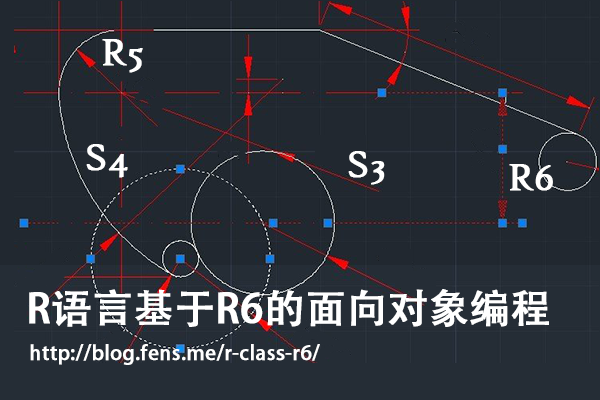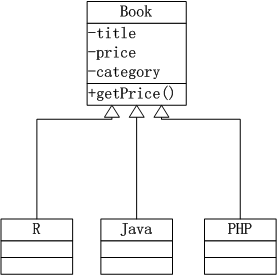• Posts tagged "R6"

# Blog Archives

## R语言基于R6的面向对象编程

R的极客理想系列文章，涵盖了R的思想，使用，工具，创新等的一系列要点，以我个人的学习和体验去诠释R的强大。

R语言作为统计学一门语言，一直在小众领域闪耀着光芒。直到大数据的爆发，R语言变成了一门炙手可热的数据分析的利器。随着越来越多的工程背景的人的加入，R语言的社区在迅速扩大成长。现在已不仅仅是统计领域，教育，银行，电商，互联网….都在使用R语言。

• 张丹(Conan), 程序员Java,R,PHP,Javascript
• weibo：@Conan_Z
• blog: http://blog.fens.me
• email: bsspirit@gmail.com

http://blog.fens.me/r-class-r6/R6是什么？听说过S3、S4和RC(R5)的面向对象类型 ，R6难道是一种新的类型吗？

1. 初识R6
2. 创建R6类和实例化对象
3. R6类的主动绑定
4. R6类的继承关系
5. R6类的对象的静态属性
6. R6类的可移植类型
7. R6类的动态绑定
8. R6类的打印函数
9. 实例化对象的存储
10. R6面向对象系统的案例

## 1. 初识R6

R6是一个单独的R包，与我们熟悉的原生的面向对象系统类型S3,S4RC类型不一样。在R语言的面向对象系统中，R6类型与RC类型是比较相似的，但R6并不基于S4的对象系统，因此我们在用R6类型开发R包的时候，不用依赖于methods包，而用RC类型开发R包的时候则必须设置methods包的依赖，在发布gridgame游戏包 文章中，就会出现RC依赖于methods包的使用情况。

R6类型比RC类型更符合其他编程对于面向对象的设置，支持类的公有成员和私有成员，支持函数的主动绑定，并支持跨包的继承关系。由于RC类型的面向对象系统设计并不彻底，所以才会有R6这样的包出现。下面就让我们体会一下，基于R6面向对象系统编程吧。

## 2. 创建R6类和实例化对象

• Win7 64bit
• R: 3.1.1 x86_64-w64-mingw32/x64 (64-bit)

``````
~ R                         # 启动R程序
> install.packages("R6")    # 安装R6包
> library(R6)               # 加载R6包
> library(pryr)             # 加载pryr包
``````

2.1 如何创建R6类？

R6对象系统是以类为基本类型， 有专门的类的定义函数 R6Class() 和 实例化对象的生成方法，下面我们用R6对象系统创建一个类。

``````
> R6Class
function (classname = NULL, public = list(), private = NULL,
active = NULL, inherit = NULL, lock = TRUE, class = TRUE,
portable = TRUE, parent_env = parent.frame())
``````

• classname 定义类名。
• public 定义公有成员，包括公有方法和属性。
• private 定义私有成员，包括私有方法和属性。
• active 主动绑定的函数列表。
• inherit 定义父类，继承关系。
• lock 是否上锁，如果上锁则用于类变量存储的环境空间被锁定，不能修改。
• class 是否把属性封装成对象，默认是封装，如果选择不封装，类中属性存存在一个环境空间中。
• portable 是否为可移植类型，默认是可移植型类，类中成员访问需要用调用self和private对象。
• parent_env 定义对象的父环境空间。

2.2 创建R6的类和实例化对象

``````
> Person <- R6Class("Person",    # 定义一个R6类
+  public=list(
+    hello = function(){         # 定义公有方法hello
+      print(paste("Hello"))
+    }
+  )
+)

> Person                   # 查看Person的定义
<Person> object generator
Public:
hello: function
Parent env:
Lock: TRUE
Portable: TRUE

> class(Person)             # 检查Person的类型
 "R6ClassGenerator"
``````

``````
> u1<-Person\$new()   # 实例化一个Person对象u1
> u1                 #查看u1对象
<Person>
Public:
hello: function
> class(u1)           # 检查u1的类型
 "Person" "R6"
``````

``````
> otype(Person)   # 查看Person类型
 "S3"
> otype(u1)       # 查看u1类型
 "S3"
``````

2.3 公有成员和私有成员

``````
> Person <- R6Class("Person",
+  public=list(
+    name=NA,                           # 公有属性
+    initialize = function(name){       # 构建函数方法
+      self\$name <- name
+    },
+    hello = function(){                # 公有方法
+      print(paste("Hello",self\$name))
+    }
+  )
+)

> conan <- Person\$new('Conan')          # 实例化对象
> conan\$hello()                         # 调用用hello()方法
 "Hello Conan"
``````

``````
> Person <- R6Class("Person",
+   public=list(                       # 公有成员
+     name=NA,
+     initialize = function(name,gender){
+       self\$name <- name
+       private\$gender<- gender        # 给私有属性赋值
+     },
+     hello = function(){
+       print(paste("Hello",self\$name))
+       private\$myGender()             # 调用私有方法
+     }
+   ),
+   private=list(                      # 私有成员
+     gender=NA,
+     myGender=function(){
+       print(paste(self\$name,"is",private\$gender))
+     }
+   )
+ )
> conan <- Person\$new('Conan','Male')         # 实例化对象
> conan\$hello()                               # 调用用hello()方法
 "Hello Conan"
 "Conan is Male"
``````

``````
> conan\$name            # 公有属性
 "Conan"
> conan\$gender          # 私有属性
NULL
> conan\$myGender()      # 私有方法
Error: attempt to apply non-function
``````

``````
> Person <- R6Class("Person",
+   public=list(
+     name=NA,
+     initialize = function(name,gender){
+       self\$name <- name
+       private\$gender<- gender
+     },
+     hello = function(){
+       print(paste("Hello",self\$name))
+       private\$myGender()
+     },
+     member = function(){              # 用于测试的方法
+       print(self)
+       print(private)
+       print(ls(envir=private))
+     }
+   ),
+   private=list(
+     gender=NA,
+     myGender=function(){
+       print(paste(self\$name,"is",private\$gender))
+     }
+   )
+ )
>
> conan <- Person\$new('Conan','Male')
> conan\$member()                            # 执行member()方法
<Person>                                    # print(self)的输出
Public:
hello: function
initialize: function
member: function
name: Conan

<environment: 0x0000000008cfc918>          # print(private)的输出
 "gender"   "myGender"                  # print(ls(envir=private))的输出
``````

## 3. R6类的主动绑定

``````
> Person <- R6Class("Person",
+   public = list(
+     num = 100
+   ),
+   active = list(                      # 主动绑定
+     active  = function(value) {
+       if (missing(value)) return(self\$num +10 )
+       else self\$num <- value/2
+     },
+     rand = function() rnorm(1)
+   )
+)

> conan <- Person\$new()
> conan\$num                   # 查看公有属性
 100
> conan\$active                # 调用主动绑定的active()函数，结果为 num +10= 100+10=100
 110
``````

``````
> conan\$active<-100    # 传参数
> conan\$num            # 查看公有属性num
 50
> conan\$active         # 调用主动绑定的active()函数，结果为 num+10=50+10=60
 60
> conan\$active(100)    # 如果进行方法调用，其实会提示没有这个函数的
Error: attempt to apply non-function
``````

``````
> conan\$rand           # 调用rand函数
 -0.4767338
> conan\$rand
 0.1063623
> conan\$rand<-99       # 传参出错
Error in (function ()  : unused argument (quote(99))
``````

## 4. R6类的继承关系

``````
> Person <- R6Class("Person",
+   public=list(                            # 公有成员
+     name=NA,
+     initialize = function(name,gender){
+       self\$name <- name
+       private\$gender <- gender
+     },
+     hello = function(){
+       print(paste("Hello",self\$name))
+       private\$myGender()
+     }
+   ),
+   private=list(                           # 私有成员
+     gender=NA,
+     myGender=function(){
+       print(paste(self\$name,"is",private\$gender))
+     }
+   )
+ )
``````

``````
> Worker <- R6Class("Worker",
+   inherit = Person,                # 继承，指向父类
+   public=list(
+     bye = function(){
+       print(paste("bye",self\$name))
+     }
+   )
+ )
``````

``````
> u1<-Person\$new("Conan","Male")        # 实例化父类
> u1\$hello()
 "Hello Conan"
 "Conan is Male"

> u2<-Worker\$new("Conan","Male")        # 实例化子类
> u2\$hello()
 "Hello Conan"
 "Conan is Male"
> u2\$bye()
 "bye Conan"
``````

``````
> Worker <- R6Class("Worker",
+   inherit = Person,
+   public=list(
+     bye = function(){
+       print(paste("bye",self\$name))
+     }
+   ),
+   private=list(
+     gender=NA,
+     myGender=function(){
+       print(paste("worker",self\$name,"is",private\$gender))
+     }
+   )
+ )
``````

``````
> u2<-Worker\$new("Conan","Male")
> u2\$hello()                    # 调用hello()方法
 "Hello Conan"
 "worker Conan is Male"
``````

``````
> Worker <- R6Class("Worker",
+   inherit = Person,
+   public=list(
+     bye = function(){
+       print(paste("bye",self\$name))
+     }
+   ),
+   private=list(
+     gender=NA,
+     myGender=function(){
+       super\$myGender()                                      # 调用父类的方法
+       print(paste("worker",self\$name,"is",private\$gender))
+     }
+   )
+ )

> u2<-Worker\$new("Conan","Male")
> u2\$hello()
 "Hello Conan"
 "Conan is Male"
 "worker Conan is Male"
``````

## 5. R6类的对象的静态属性

``````
> A <- R6Class("A",
+  public=list(
+    x = NULL
+  )
+ )
>
> B <- R6Class("B",
+  public = list(
+    a = A\$new()
+  )
+ )
``````

``````
> b <- B\$new()         # 实例化B对象
> b\$a\$x <- 1           # 给x变量赋值
> b\$a\$x                # 查看x变量的值
 1

> b2 <- B\$new()        # 实例化b2对象
> b2\$a\$x <- 2          # 给x变量赋值
> b2\$a\$x               # 查看x变量的值
 2

> b\$a\$x                # b实例的a对象的x值也发生改变
 2
``````

``````
> C <- R6Class("C",
+  public = list(
+    a = NULL,
+    initialize = function() {
+      a <<- A\$new()
+    }
+  )
+ )

> cc <- C\$new()
> cc\$a\$x <- 1
> cc\$a\$x
 1

> cc2 <- C\$new()
> cc2\$a\$x <- 2
> cc2\$a\$x
 2

> cc\$a\$x        # x值未发生改变
 1
``````

## 6. R6类的可移植类型

• 可移植类型支持跨R包的继承；不可移植类型，在跨R包继承的时候，兼容性不太好。
• 可移植类型必须要用self对象和private对象来访问类中的成员，如self\$x,private\$y；不可移植类型，可以直接使用变量x,y，并通过<<-实现赋值。

``````
> RC <- setRefClass("RC",                  # RC类型的定义
+   fields = list(x = 'Hello'),
+   methods = list(
+     getx = function() x,
+     setx = function(value) x <<- value
+   )
+ )
> rc <- RC\$new()
> rc\$setx(10)
> rc\$getx()
 10
``````

``````
> NR6 <- R6Class("NR6",                # R6不可移植类型
+   portable = FALSE,
+   public = list(
+     x = NA,
+     getx = function() x,
+     setx = function(value) x <<- value
+   )
+ )
> np6 <- NR6\$new()
> np6\$setx(10)
> np6\$getx()
 10
``````

``````
> PR6 <- R6Class("PR6",
+   portable = TRUE,            # R6可移植类型
+   public = list(
+    x = NA,
+    getx = function() self\$x,
+    setx = function(value) self\$x <- value
+   )
+ )
> pr6 <- PR6\$new()
> pr6\$setx(10)
> pr6\$getx()
 10
``````

## 7. R6类的动态绑定

R6包已考虑这个情况，提供了一种动态设置成员变量的方法用\$set()函数。

``````
> A <- R6Class("A",
+   public = list(
+     x = 1,
+     getx = function() x
+   )
+ )
> A\$set("public", "getx2", function() self\$x*2)     # 动态增加getx2()方法
> s <- A\$new()
> s                     # 查看实例化对象的结构
<A>
Public:
getx: function
getx2: function
x: 1
> s\$getx2()             # 调用getx2()方法
 20
``````

``````
> A\$set("public", "x", 10, overwrite = TRUE)     # 动态改变x属性
> s <- A\$new()
> s\$x                                            # 查看x属性
 10
> s\$getx()                                       # 调用getx()方法，可移植类型x变量丢失
``````

``````
> A <- R6Class("A",
+  public = list(
+    x = 1,
+    getx = function() self\$x     # 修改为self\$x
+  )
+ )
> A\$set("public", "x", 10, overwrite = TRUE)
> s <- A\$new()
> s\$x
 10
> s\$getx()                        # 调用getx()方法
 10
``````

## 8. R6类的打印函数

R6提供了用于打印的默认方法print()，每当要打印实例化对象时，都会调用这个默认的print()方法，有点类似于Java类中默认的toString()方法。

``````
> A <- R6Class("A",
+  public = list(
+    x = 1,
+    getx = function() self\$x
+  )
+ )
> a <- A\$new()
> print(a)             # 使用默认的打印方法
<A>
Public:
getx: function
x: 1
``````

``````
> A <- R6Class("A",
+    public = list(
+      x = 1,
+      getx = function() self\$x,
+      print = function(...) {
+        cat("Class <A> of public ", ls(self), " :", sep="")
+        cat(ls(self), sep=",")
+        invisible(self)
+      }
+    )
+ )
> a <- A\$new()
> print(a)
Class <A> of public getxprintx :getx,print,x
``````

## 9. 实例化对象的存储

R6是基于S3面向对象系统的构建，而S3类型又是一种比较松散的类型，会造成用户环境空间的变量泛滥的问题。R6提供了一种方式，设置R6Class()的class参数，把类中定义的属性和方法统一存储到一个S3对象中，这种方式是默认的。另一种方式为，把把类中定义的属性和方法统一存储到一个单独的环境空间中。

``````
> A <- R6Class("A",
+  class=TRUE,
+  public = list(
+    x = 1,
+    getx = function() self\$x
+  )
+ )
> a <- A\$new()
> class(a)
 "A"  "R6"
> a
<A>
Public:
getx: function
x: 1
``````

``````
> B <- R6Class("B",
+   class=FALSE,
+   public = list(
+     x = 1,
+     getx = function() self\$x
+   )
+ )
> b <- B\$new()
> class(b)
 "environment"
> b
<environment: 0x000000000d83c970>
> ls(envir=b)
 "getx" "x"
``````

``````
> A <- R6Class("A",
+   lock=TRUE,       # 锁定环境空间
+   public = list(
+     x = 1
+   )
+ )
> s<-A\$new()
> ls(s)         # 查看s环境空间的变量
 "x"
> s\$aa<-11      # 增加新变量，错误
Error in s\$aa <- 11 : cannot add bindings to a locked environment
> rm("x",envir=s)       # 删除原有变量，错误
Error in rm("x", envir = s) :
cannot remove bindings from a locked environment
``````

``````
> A <- R6Class("A",
+  lock=FALSE,         # 不锁定环境空间
+  public = list(
+    x = 1
+  )
+ )
> s<-A\$new()
> ls(s)         # 查看s环境空间的变量
 "x"
> s\$aa<-11      # 增加变量
> ls(s)
 "aa" "x"
> rm("x",envir=s)    # 删除变量
> ls(s)
 "aa"
``````

## 10. R6面向对象系统的案例``````
> Book <- R6Class("Book",            # 父类
+    private = list(
+      title=NA,
+      price=NA,
+      category=NA
+    ),
+   public = list(
+     initialize = function(title,price,category){
+       private\$title <- title
+       private\$price <- price
+       private\$category <- category
+     },
+     getPrice=function(){
+       private\$price
+     }
+   )
+ )

> R <- R6Class("R",     # 子类R图书
+    inherit = Book
+ )
> Java <- R6Class("JAVA",  # 子类JAVA图书
+   inherit = Book
+ )
> Php <- R6Class("PHP",    # 子类PHP图书
+   inherit = Book
+ )
``````

``````
> r1<-R\$new("R的极客理想-工具篇",59,"R")
> r1\$getPrice()
 59

> j1<-Java\$new("Java编程思想",108,"JAVA")
> j1\$getPrice()
 108

> p1<-Java\$new("Head First PHP & MySQL",98,"PHP")
> p1\$getPrice()
 98
``````

• 所有图书9折
• JAVA图书7折，不支持重复打折
• 为了推动R图书的销售，R语言图书7折，并支持重复打折
• PHP图书无特别优惠

``````
> Book <- R6Class("Book",
+   private = list(
+     title=NA,
+     price=NA,
+     category=NA
+   ),
+   public = list(
+     initialize = function(title,price,category){
+       private\$title <- title
+       private\$price <- price
+       private\$category <- category
+     },
+     getPrice=function(){
+       p<-private\$price*self\$discount()
+       print(paste("Price:",private\$price,", Sell out:",p,sep=""))
+     },
+     discount=function(){
+       0.9
+     }
+   )
+ )
``````

3个子类，分别对应自己的打折规则，分别进行修改。

• 给JAVA子类增加 discount()方法，用于覆盖父类的discount()方法，让JAVA图书7折，不支持重复打折，从而满足第二条规则。
• 给R子类增加 discount()方法，在子类的discount()方法中调用父类的discount()方法，让支持 R图书7折和9折的折上折，从而满足第三条规则。
• PHP子类，没有修改，完全遵循第一条规则的。
• ``````
> Java <- R6Class("JAVA",
+   inherit = Book,
+   public = list(
+     discount=function(){
+       0.7
+     }
+   )
+ )
>
> R <- R6Class("R",
+   inherit = Book,
+   public = list(
+     discount=function(){
+       super\$discount()*0.7
+     }
+   )
+ )
>
> Php <- R6Class("PHP",
+   inherit = Book
+ )
``````

分别查看3本图书的折后价格。

``````
> r1<-R\$new("R的极客理想-工具篇",59,"R")
> r1\$getPrice()
 "Price:59, Sell out:37.17"   # 59 * 0.9 *0.7= 37.17
>
> j1<-Java\$new("Java编程思想",108,"JAVA")
> j1\$getPrice()
 "Price:108, Sell out:75.6"    # 108 *0.7= 75.6
>
> p1<-Php\$new("Head First PHP & MySQL",98,"PHP")
> p1\$getPrice()
 "Price:98, Sell out:88.2"      # 98 *0.9= 88.2
``````

R图书打折最多，享受7折和9折的折上折优惠， 59 * 0.9 * 0.7= 37.17；Java图书享受7折优惠，108 *0.7= 75.6；PHP图书享受9折优惠 98 *0.9= 88.2。

通过这个实例，我们用R6的方法实现了面向对象编程中的封装、继承和多态的3个特征，证明R6是一种完全的面向对象的实现。R6类对象系统，提供了一种可兼容的面向对象实现方式，更接近于其他的编程语言上的面向对象的定义，由于R6底层基于S3来实现的，所以比RC的类更加有效果。

我们一共介绍了4种R语言的面向对象体系结构，选择自己理解的，总有一种会适合你。

转载请注明出处：
http://blog.fens.me/r-class-r6/